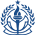## Tuesday, September 6, 2016

### Riemann Summation and Physics Simulation are Statistically Biased

Riemann summation is the simplest method for numerically approximating integrals. in which you chop up the domain into equally sized segments and add a bunch of rectangles together.

$$\int f(x) dx \sim \sum_i^k f(x) \Delta x$$

This technique also comes up in Euler integration for simulating physics:

\begin{align*} \Delta x & = \int \dot{x}(t) dt \\ & \sim \dot{x}(t) \Delta t \end{align*}

These are all special cases of "Deterministic Quadratures", where the integration step size $\Delta x$ is non-random ("deterministic") and we are summing up a bunch of quadrilateral pieces ("Quadratures") to approximate areas.

This post interprets the numerical integration as a special case of stratified sampling, and shows that deterministic quadrature rules are statistically biased estimators.

Suppose we want to compute $E_X[f(X)] = \int_x p(x)\cdot f(x) dx$ via a Monte Carlo estimator.

A plain Monte Carlo Estimator is given by $\mu = \frac{1}{n}\sum_i^n f(X_i)$, and has variance $\frac{1}{n}\sigma^2$ where $\sigma^2=Var[X_i]$.

Stratified sampling (see previous blog post) introduces a stratification variable $Y$ with a discrete distribution of $k$ strata with probability densities  $p(y_1),p(y_2),...,p(y_k)$, respectively. A common choice is to assign each $p(y_1) = p(y_2) = ... = p(y_k) = 1/k$, and arrange the strata in a grid. In this case, $y_i$ correspond to the corners of the strata and $P(X|Y=y_i)$ corresponds to a uniform distribution over that square ($X_i = Y_i + 0.1*\text{rand}()$).

The variance of this estimator is $\sum_i^k p(y_i)^2 \cdot V_i$, where $V_i$ is the variance of $\mathbb{E}[X|Y=y_i]$ for strata $i$.

Suppose we let $\mathbb{E}[X|Y=y_i]$ as $y_i$ - that is, we just sample at the corners where $y_i$ sampels are.

The good news is that $V_i = 0$, and we've reduced the variance of the total estimator to zero. The bad news is that the estimator is now biased because our per-strata estimators $\mathbb{E}[X|Y=y_i]$ are biased (our estimator never uses information from the interiors/edges of the squares). The estimator is also inconsistent for finite strata.

Does this figure remind you of anything? This estimator behaves identically to a Riemann Summation in 2D!

What does this mean?

• If you ever take a Riemann sum with fixed step size, your integral is biased!
• If you are monitoring mean ocean temperatures and place your sensors in an regular grid across the water (like above), your estimator is biased!
• If you are recording video at a too-low frequency and pick up aliasing artifacts, your estimates are biased!
• If you use a fixed timestep in a physics simulation, such as Euler or Runge-Kutta methods, your space integrals are biased!

The takeaway here is that *all* deterministic quadrature rules are statistically biased. that's okay though - the expected value of biased estimators *do* converge to the true expectation as you crank the number of strata to infinity (e.g. $\Delta x \to 0$).

A "less-biased" physics simulator ought to incorporate random time steps (for instance, sampled between 0 and MAX_TIME_STEP). To reduce variance, one might generate multiple samples, compute a nonlinear update, then average out the integration.

Fairly obvious in hindsight, but I found it interesting to think about the relationship between stratified sampling and deterministic quadrature :)

1.2.